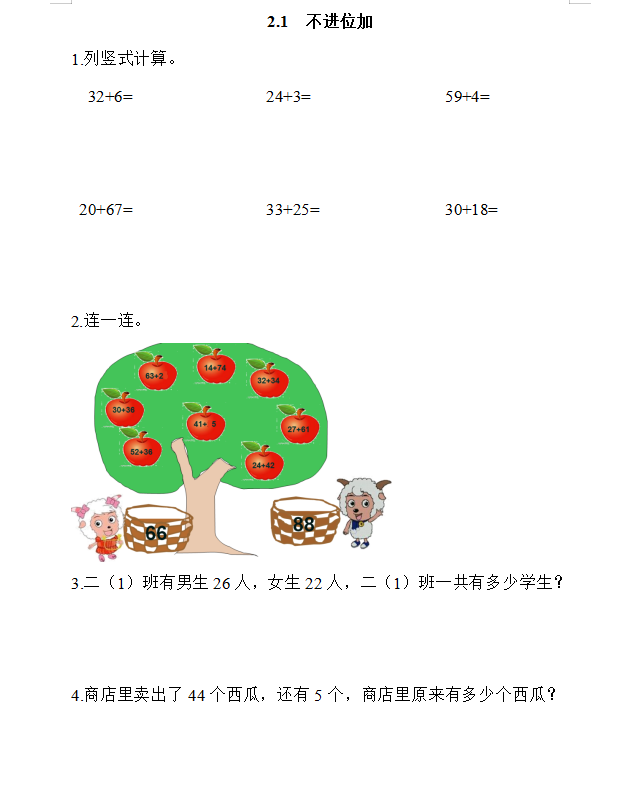2.1  不进位加

1.列竖式计算。

32+6=                24+3=                59+4=

20+67=                33+25=               30+18=